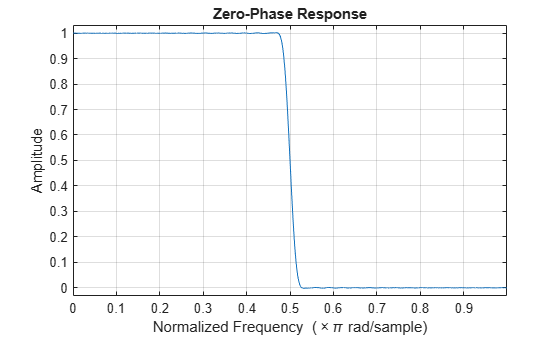# zerophase

Zero-phase response of discrete-time filter System object

## Syntax

``````[zphase,w] = zerophase(sysobj)``````
``````[zphase,w] = zerophase(sysobj,n)``````
``````[zphase,w] = zerophase(___,'Arithmetic',arithType)``````
``zerophase(sysobj)``

## Description

``````[zphase,w] = zerophase(sysobj)``` returns the zero-phase response vector, `zphase`, and the corresponding frequency vector, `w`, in radians/sample of the filter System object™ based on the current filter coefficients.```
``````[zphase,w] = zerophase(sysobj,n)``` returns the `n`-point zero-phase response vector and the corresponding `n`-point frequency vector in radians/sample of the filter System object.```
``````[zphase,w] = zerophase(___,'Arithmetic',arithType)``` analyzes the filter System object based on the arithmetic specified in `arithType` using either of the previous syntaxes.```

example

````zerophase(sysobj)` launches `fvtool` and shows the zero-phase response of the filter System object.For more input options, see `zerophase` in Signal Processing Toolbox™.```

## Examples

collapse all

Create a dsp.FIRFilter System object™ and compute the zero-phase response of the filter.

```Fs = 8000; Fcutoff = 2000; FIRFilt = dsp.FIRFilter('Numerator', fir1(130,Fcutoff/(Fs/2)));```

The `zerophase` function computes the zero-phase response of the filter and displays the response using `fvtool`.

`zerophase(FIRFilt)`## Input Arguments

collapse all

Number of points over which the zero-phase response is evaluated around the upper half of the unit circle. For an FIR filter where `n` is a power of two, the computation is done faster using FFTs.

Data Types: `double`

Arithmetic used in the filter analysis, specified as `'double'`, `'single'`, or `'Fixed'`. When the arithmetic input is not specified and the filter System object is unlocked, the analysis tool assumes a double-precision filter. When the arithmetic input is not specified and the System object is locked, the function performs the analysis based on the data type of the locked input.

The `'Fixed'` value applies to filter System objects with fixed-point properties only.

When the `'Arithmetic'` input argument is specified as `'Fixed'` and the filter object has the data type of the coefficients set to `'Same word length as input'`, the arithmetic analysis depends on whether the System object is unlocked or locked.

• unlocked –– The analysis object function cannot determine the coefficients data type. The function assumes that the coefficients data type is signed, has a 16-bit word length, and is auto scaled. The function performs fixed-point analysis based on this assumption.

• locked –– When the input data type is `'double'` or `'single'`, the analysis object function cannot determine the coefficients data type. The function assumes that the data type of the coefficients is signed, has a 16-bit word length, and is auto scaled. The function performs fixed-point analysis based on this assumption.

To check if the System object is locked or unlocked, use the `isLocked` function.

When the arithmetic input is specified as `'Fixed'` and the filter object has the data type of the coefficients set to a custom numeric type, the object function performs fixed-point analysis based on the custom numeric data type.

## Output Arguments

collapse all

Zero-phase response vector, evaluated at `n` equally-spaced points around the upper half of the unit circle, returned as a vector. If `n` is not specified, the function uses a default value of `8192`.

Data Types: `double`

Frequency vector of length `n` in radians/sample. `w` consists of `n` equally-spaced points around the upper half of the unit circle (from 0 to π radians/sample). If `n` is not specified, the function uses a default value of `8192`.

Data Types: `double`

Introduced in R2011a

## SupportGet trial now